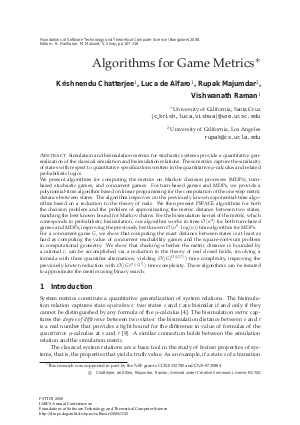Document# Algorithms for Game Metrics

### Authors Krishnendu Chatterjee, Luca de Alfaro, Rupak Majumdar, Vishwanath Raman## File

LIPIcs.FSTTCS.2008.1745.pdf
• Filesize: 431 kB
• 12 pages

## Cite As

Krishnendu Chatterjee, Luca de Alfaro, Rupak Majumdar, and Vishwanath Raman. Algorithms for Game Metrics. In IARCS Annual Conference on Foundations of Software Technology and Theoretical Computer Science. Leibniz International Proceedings in Informatics (LIPIcs), Volume 2, pp. 107-118, Schloss Dagstuhl - Leibniz-Zentrum für Informatik (2008)
https://doi.org/10.4230/LIPIcs.FSTTCS.2008.1745

## Abstract

Simulation and bisimulation metrics for stochastic systems provide a quantitative generalization of the classical simulation and bisimulation relations. These metrics capture the similarity of states with respect to quantitative specifications written in the quantitative $\mu$-calculus and related probabilistic logics. We present algorithms for computing the metrics on Markov decision processes (MDPs), turn-based stochastic games, and concurrent games. For turn-based games and MDPs, we provide a polynomial-time algorithm based on linear programming for the computation of the one-step metric distance between states. The algorithm improves on the previously known exponential-time algorithm based on a reduction to the theory of reals. We then present PSPACE algorithms for both the decision problem and the problem of approximating the metric distance between two states, matching the best known bound for Markov chains. For the bisimulation kernel of the metric, which corresponds to probabilistic bisimulation, our algorithm works in time $\calo(n^4)$ for both turn-based games and MDPs; improving the previously best known $\calo(n^9\cdot\log(n))$ time algorithm for MDPs. For a concurrent game $G$, we show that computing the exact distance between states is at least as hard as computing the value of concurrent reachability games and the square-root-sum problem in computational geometry. We show that checking whether the metric distance is bounded by a rational $r$, can be accomplished via a reduction to the theory of real closed fields, involving a formula with three quantifier alternations, yielding $\calo(|G|^{\calo(|G|^5)})$ time complexity, improving the previously known reduction with $\calo(|G|^{\calo(|G|^7)})$ time complexity. These algorithms can be iterated to approximate the metrics using binary search.
##### Keywords
• Algorithms
• Metrics
• Kernel
• Simulation
• Bisimulation
• Linear Programming
• Theory of Reals

## Metrics

• Access Statistics
• Total Accesses (updated on a weekly basis)
0